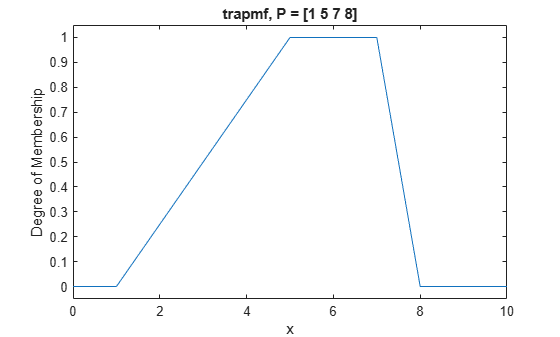Documentation

# trapmf

Trapezoidal membership function

This function computes fuzzy membership values using a trapezoidal membership function. You can also compute this membership function using a `fismf` object. For more information, see fismf Object.

This membership function is related to the `trimf` membership function.

## Syntax

``y = trapmf(x,params)``

## Description

example

````y = trapmf(x,params)` returns fuzzy membership values computed using the following trapezoidal membership function:$f\left(x;a,b,c,d\right)=\left\{\begin{array}{cc}0,& x\le a\\ \frac{x-a}{b-a},& a\le x\le b\\ 1,& b\le x\le c\\ \frac{d-x}{d-c},& c\le x\le d\\ 0,& d\le x\end{array}\right\}$or, more compactly: $f\left(x;a,b,c,d\right)=\mathrm{max}\left(\mathrm{min}\left(\frac{x-a}{b-a},1,\frac{d-x}{d-c}\right),o\right)$To specify the parameters, a, b, c, and d, use `params`.Membership values are computed for each input value in `x`.```

## Examples

collapse all

```x = 0:0.1:10; y = trapmf(x,[1 5 7 8]); plot(x,y) xlabel('trapmf, P = [1 5 7 8]') ylim([-0.05 1.05])```## Input Arguments

collapse all

Input values for which to compute membership values, specified as a scalar or vector.

Membership function parameters, specified as the vector [a b c d]. Parameters b and c define the shoulders of the membership function, and a and d define its feet.

## Output Arguments

collapse all

Membership value returned as a scalar or a vector. The dimensions of `y` match the dimensions of `x`. Each element of `y` is the membership value computed for the corresponding element of `x`.

## Alternative Functionality

### `fismf` Object

You can create and evaluate a `fismf` object that implements the `trapmf` membership function.

```mf = fismf("trapmf",P); Y = evalmf(mf,X);```

Here, `X`, `P`, and `Y` correspond to the `x`, `params`, and `y` arguments of `trapmf`, respectively.

Download now